output.to from Sideway

Fluid Kinematics

Draft for Information Only

# Content

`Fluid Kinematics Velocity Field Acceleration Field`

# Fluid Kinematics

Kinematics is the study of the velocity and acceleration of the fluid motion. There are two methods to study the motion of fluid. One way can focus on fluid particles by tracking their position vector, i.e.and determining their associated properties as a function of time, i.e. velocity vector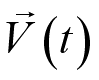. This is the Lagrangian description of fluid motion. The other way of describing fluid motion is the Eulerian description of fluid motion, which focuses on the fluid flows in and out of a control volume in terms of field variables. Instead of following individual fluid particles, the description describes the variables value of  the location only and does not care whichever fluid particle occupying that location inside the control volume  as a function of space and time, i.e.  pressure field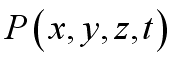, velocity fieldto define the flow field.

## Velocity Field

Fluid property can be represented as a function of spatial coordinates in term of a field representation. For a fluid flow, it is also a function of time.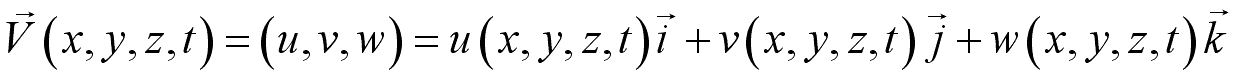When the velocity value of a location equals to zero,i.e., it is called a stagnation point.

## Acceleration Field

In a fluid flow, the velocity of a particle is a function of its location and time. Imply. By definition, the acceleration of a particle is the time rate of change of its velocity,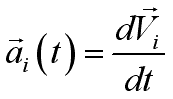. Using chain rule of differentiation,and because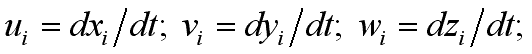imply.

Since the equation can be used to describe any particle, the acceleration field can be obtained from the velocity field as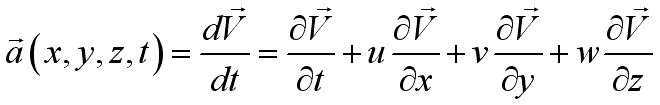,or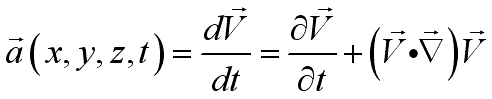;
whereis named local acceleration or unsteady acceleration, which equals to zero for steady flow and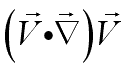is named advective acceleration or convective acceleration, which equals to non-zero even for steady flow.

And the total acceleration can be expressed as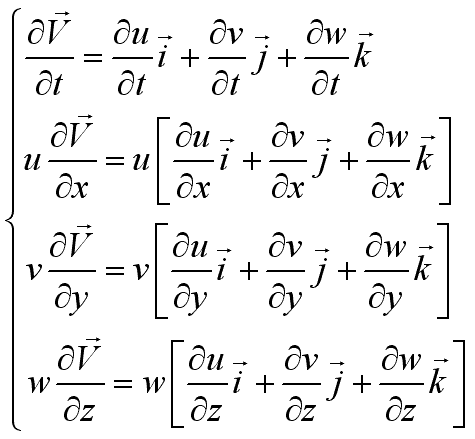orSince the total derivative operatoris derived from a fluid particle, it is called material derivative,. i.e.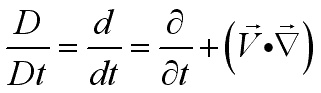The total acceleration is therefore also called material acceleration.

©sideway

ID: 100100002 Last Updated: 2/7/2010 Revision: 1Home (5)

Business

Management

HBR (3)

Information

Recreation

Hobbies (7)

Culture

Chinese (1097)

English (336)

Reference (66)

Computer

Hardware (149)

Software

Application (187)

Digitization (24)

Numeric (19)

Programming

Web (648)CSS (SC)

ASP.NET (SC)

HTML

Knowledge Base

Common Color (SC)

Html 401 Special (SC)

OS (389)

MS Windows

Windows10 (SC)

.NET Framework (SC)

DeskTop (7)

Knowledge

Mathematics

Formulas (8)

Number Theory (206)

Algebra (20)

Trigonometry (18)

Geometry (18)

Calculus (67)

Complex Analysis (21)

Engineering

Tables (8)

Mechanical

Mechanics (1)

Rigid Bodies

Statics (92)

Dynamics (37)

Fluid (5)

Fluid Kinematics (5)

Control

Acoustics (19)

Biology (1)

Geography (1)

Copyright © 2000-2019 Sideway . All rights reserved Disclaimers last modified on 10 Feb 2019